you are here : home » portfolio » Resident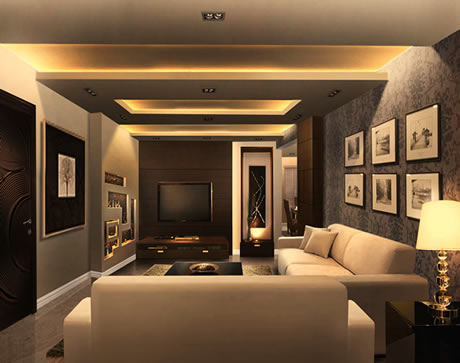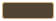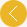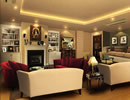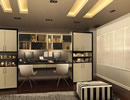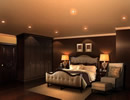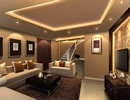This is the caption of image number 1
This is the caption of image number 2
This is the caption of image number 3
This is the caption of image number 4
This is the caption of image number 5
This is the caption of image number 6
This is the caption of image number 7
This is the caption of image number 8
This is the caption of image number 9
This is the caption of image number 10
This is the caption of image number 11
This is the caption of image number 12
This is the caption of image number 13
This is the caption of image number 14